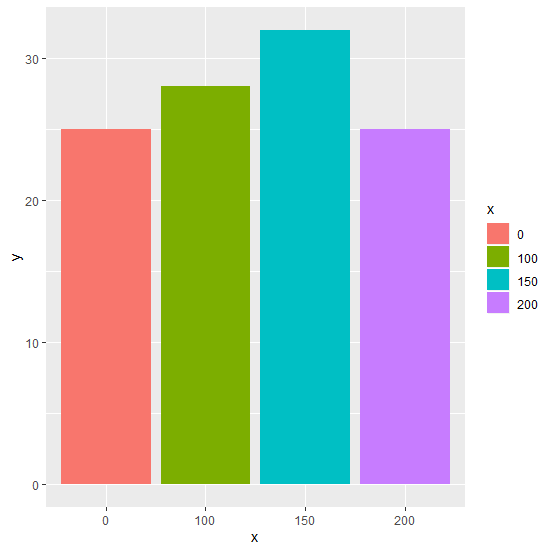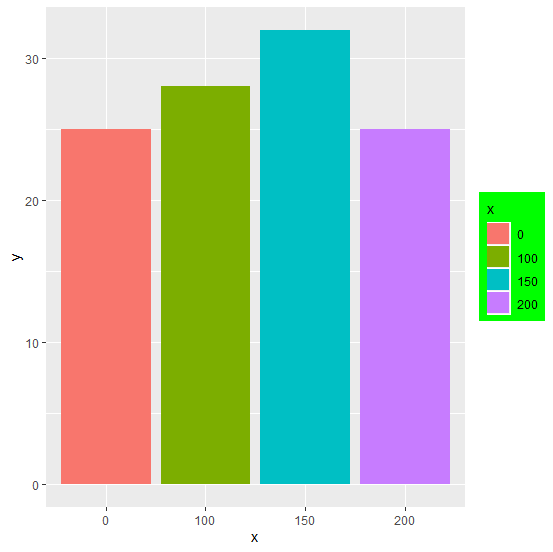# How to display the legend of a bar plot in a colored box in R?

When we create a bar plot or any other plot with legend, the background of the legend is white but it can be changed to any color with the help of scales package. We can make changes in the legend of a plot using alpha in legend.background argument of theme function. This will help us to change the background color of the legend.

## Example

Live Demo

> x<-c("0","100","150","200")
> y<-c(25,28,32,25)
> df<-data.frame(x,y)
> df

## Output

   x  y
1   0 25
2 100 28
3 150 32
4 200 25

Creating a bar plot with legend −

> library(ggplot2)
> ggplot(df,aes(x,y,fill=x))+geom_bar(stat="identity")

## OutputChanging the background color of the legend −

> library(scales)
> ggplot(df,aes(x,y,fill=x))+geom_bar(stat="identity")+theme(legend.background=element
_rect(fill=alpha("green")))

## Output